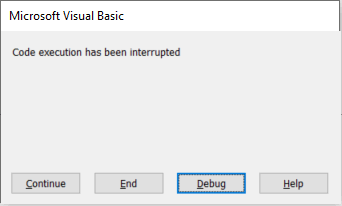# Usage of for, while, for each, do loops in Visual Basic

Loops in programming is a technique to iterate over the elements of collections and arrays and other data sets.

The following sections explains different types of loops. All the below snippets based on a simple array of String which is declared below:

```Dim Arr(9) As String

Sub InitArr()
Arr(0) = "A": Arr(1) = "B": Arr(2) = "C": Arr(3) = "D": Arr(4) = "E"
Arr(5) = "F": Arr(6) = "G": Arr(7) = "H": Arr(8) = "I": Arr(9) = "J"
End Sub
```

However similar technique can be used with any type of arrays.

## For-Next

This is probably the most common loop type. It allows to perform specified number of iterations. By default each loop iteration will increment the value of index by one.

```Sub ForLoop()

InitArr

Dim i As Integer

For i = 0 To 9
Dim val As String
val = Arr(i)
Debug.Print val
Next

End Sub
```

The above sample will print all values from the array:

A B C D E F G H I J

Alternatively, different value for step can be specified with the Step keyword. Step can be negative to iterate in reverse direction.

```Sub ForLoopStep()

InitArr

Dim i As Integer

For i = UBound(Arr) To 0 Step -1
Dim val As String
val = Arr(i)
Debug.Print val
Next

End Sub
```

The above code would output values in the reverse direction:

J I H G F E D C B A

## While-Wend

This loop executes while the condition is True

This type of loop can be useful when number of iterations is not known in advance.

```Sub WhileLoop()

InitArr

Dim i As Integer
Dim val As String
i = 0

While val <> "D"
val = Arr(i)
i = i + 1
Debug.Print val
Wend

End Sub
```

The above code would output and terminates when the current element equals to D:

A B C D

## Do-Loop While

Do-Loop While are similar to While-Wend loops with an exception that the condition executed after the step, so it ensures that at least one iteration will be performed regardless of the condition

```Sub DoLoop()

InitArr

Dim i As Integer
Dim val As String
i = 0

Do
val = Arr(i)
i = i + 1
Debug.Print val
Loop While val <> "D"

End Sub
```

The above code would produce:

A B C D

## For Each-Next

Although in most cases For-Next loop is used to iterate over the elements of an array it is not restricted to this use case only. Any code can be executed within the body of the loop.

To specifically iterate over the elements of an array For Each-Next loop can be used

```Sub ForEachLoop()

InitArr

For Each x In Arr
Debug.Print x
Next

End Sub
```

The above snippet would output:

J I H G F E D C B A

## Infinite Loops

Incorrect use of loops conditions may result into infinite loop. Such code usually results in the software hang or crash.

For example the below loop would run infinitely as i variable is never incremented.

```Sub InifiniteLoop()

InitArr

Dim i As Integer
i = 0

While i <> UBound(Arr) + 1
Debug.Print Arr(i)
Wend

End Sub
```

To terminate the infinite loop (or any running code in VBA) it is possible to press the combination of keys: ctrl+alt+pause/break

This would display the below message box and code can be stopped or entered into debug mode.Terminating infinite loop